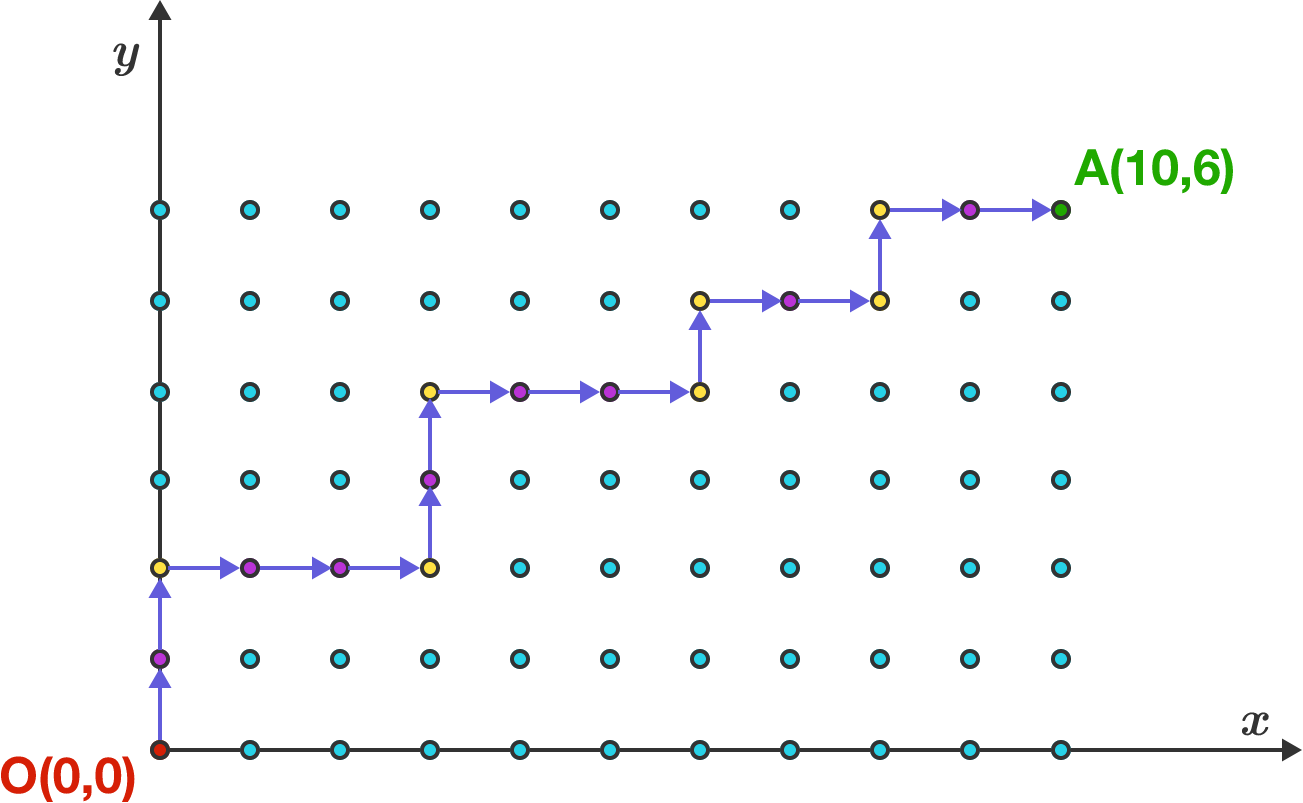# Feynman Checkerboard!Richard P Feynman starts his journey from $O=(0,0)$ towards $A=(10,6).$ He can only go in the $+x$ and $+y$ directions along the lattice, and he must turn exactly 7 times. An example path is shown above, with the yellow dots indicating turns.

How many ways can Feynman travel from $O$ to $A$ in this way?

Bonus: Consider the general case, traveling from $(0,0)$ to $(p,q)$ with exactly $r$ turns.

×